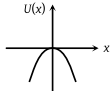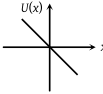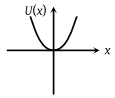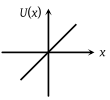A particle is placed at the origin and a force F = kx is acting on it (where k is positive constant). If U(0) = 0, the graph of U(x) versus x will be (where U is the potential energy function)

(1)(2)(3)(4)Concept Questions :-

Elastic potential energy
High Yielding Test Series + Question Bank - NEET 2020

Difficulty Level:

A particle moves from a point $\left(-2\stackrel{^}{i}+5\stackrel{^}{j}\right)$ to $\left(4\stackrel{^}{j}+3\stackrel{^}{k}\right)$ when a force of  $\left(4\stackrel{^}{i}+3\stackrel{^}{j}\right)$ N is applied. How much work has been done by the force?

1. 8 J

2. 11 J

3. 5 J

4. 2 J

Concept Questions :-

Concept of work
High Yielding Test Series + Question Bank - NEET 2020

Difficulty Level:

A body of mass 1 kg begins to move under the action of a time dependent force  N, where $\stackrel{^}{i}$ and $\stackrel{^}{j}$ are unit vectors along X and Y axis, What power will be developed by the force at the time (t) ?

(a) $\left(2{t}^{2}+4{t}^{4}\right)W$          (b) $\left(2{t}^{3}+3{t}^{4}\right)W$

(c) $\left(2{t}^{3}+3{t}^{5}\right)W$          (d) $\left(2t+3{t}^{3}\right)W$

Concept Questions :-

Power
High Yielding Test Series + Question Bank - NEET 2020

Difficulty Level:

Two similar springs P and Q have spring constants KP and KQ, such that KP > KQ. They are stretched, first by the same amount (case a) and then by the same force (case b). The work done by external force, WP and WQ on the springs P and Q in case (a) and case (b) respectively are related as,

1. WP=WQ ; WP>WQ

2. WP=WQ ; WP=WQ

3. WP>WQ ; WQ>WP

4. WP<WQ ; WQ<WP

Concept Questions :-

Elastic potential energy
High Yielding Test Series + Question Bank - NEET 2020

Difficulty Level:

A block of mass 10 kg, moving in the x-direction with a constant speed of 10 ms-1, is subjected to a retarding force F=0.1x J/m during its travel from x=20m to 30m. Its final KE will be :

(a) 475 J
(b) 450 J
(c) 275 J
(d) 250 J

Concept Questions :-

Work done by variable force
High Yielding Test Series + Question Bank - NEET 2020

Difficulty Level:

A particle of mass m is driven by a machine that delivers a constant power of k watts. If the particle starts from rest the force on the particle at time t is:
(a) $\sqrt{\left(\frac{mk}{2}\right)}{t}^{-1/2}$

(b) $\sqrt{\left(mk\right)}{t}^{-1/2}$

(c) $\sqrt{\left(2mk\right)}{t}^{-1/2}$

(d) $\frac{1}{2}\sqrt{\left(mk\right)}{t}^{-1/2}$

Concept Questions :-

Power
High Yielding Test Series + Question Bank - NEET 2020

Difficulty Level:

Two particles of masses m1,m2 move with initial velocities u1 and u2. On collision, one of the particles get excited to higher level, after absorbing energy $\epsilon$. If final velocities of particles be v1 and v2, then we must have

(a)m12u1+m22u2-$\epsilon$=m12v1+m22v2

(b)$\frac{1}{2}$m1u12+$\frac{1}{2}$m2u2=$\frac{1}{2}$m1v12+$\frac{1}{2}$m2v22-$\epsilon$

(c)$\frac{1}{2}$m1u12+$\frac{1}{2}$m2u22-$\epsilon$=$\frac{1}{2}$m1v12+$\frac{1}{2}$m2v22

(d)$\frac{1}{2}$m12u12+$\frac{1}{2}$m22u22+$\epsilon$=$\frac{1}{2}$m12v12+$\frac{1}{2}$m22v22

Concept Questions :-

Work-Energy theorem
High Yielding Test Series + Question Bank - NEET 2020

Difficulty Level:

A ball is thrown vertically downwards from a height of 20m with an initial velocity vo . It collides with the ground, loses 50% of it's energy in collision and rebounds to the same height. The initial velocity vo is (Take, g = 10 ms-2)

(a) 14 ms-1

(b) 20ms-1

(c) 28ms-1

(d) 10ms-1

Concept Questions :-

Gravitational Potential energy
High Yielding Test Series + Question Bank - NEET 2020

Difficulty Level:

A uniform force of (3i + j) N acts on a particle of mass 2 kg. Hence the particle is displaced from position (2i+k) m to position (4i+3j-k) m. The work done by the force on the particle is-

(a) 9J

(b) 6J

(c) 13J

(d) 15J

Concept Questions :-

Concept of work
High Yielding Test Series + Question Bank - NEET 2020

Difficulty Level:

A body of mass m is taken from the earth’s surface to the height equal to twice the radius (R) of the earth. The change is potential energy of body will be

(a)mg2R

(b)2/3mgR

(c)3mgR

(d)1/3mgR

Concept Questions :-

Gravitational Potential energy